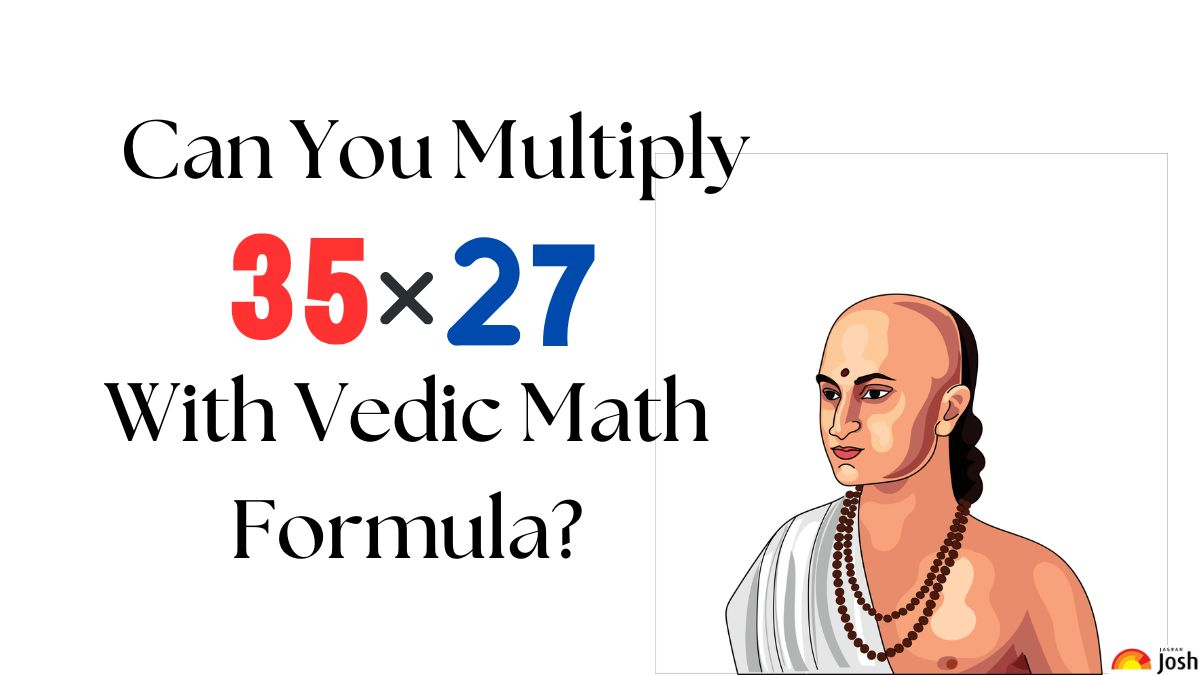# Vedic Math Puzzle: Can You Solve this High IQ Vedic Math Equation in 21 Seconds? Try Your Skills!

Vedic Math Puzzle: Sharpen up your mental math abilities, leading to improved computational speed and efficiency with this Vedic Math Puzzle. Hurry Up!Do you know how to solve the Vedic Math Equation?

Vedic Math Puzzle: Vedic mathematics is an ancient Indian system of mathematics that originated from the Vedas, the ancient scriptures of India. Vedic math puzzles are an intriguing aspect of this system that can enhance mathematical skills while providing an enjoyable and challenging experience.

Look for the equation below:

Do You Think You Will Be Able To Solve?

Don’t Say It’s Not Your Cup Of Tea!

How does Vedic Math work?

Vedic math puzzles introduce learners to unique mathematical concepts and principles that go beyond traditional arithmetic methods. One of the key advantages of Vedic math puzzles is their ability to enhance mental calculation skills. These puzzles often involve finding shortcuts and patterns in mathematical calculations, allowing individuals to perform complex calculations quickly and accurately in their heads. By engaging in Vedic math puzzles regularly, learners can sharpen their mental math abilities, leading to improved computational speed and efficiency.

Only 1% Of Genius Can Find The Product of The Two Digits Below With Vedic Sutra in 19 Seconds. Hurry Up!

Hey Genius…

You are Losing…

These puzzles often present challenges that require learners to break down complex problems into simpler components, encouraging them to develop problem-solving strategies and think outside the box. By engaging with Vedic math puzzles, individuals can strengthen their analytical and logical thinking skills, which are essential not only in mathematics but also in various real-life scenarios.

Tick…

Tock…

Tick…

The answer for the tricky Vedic math equation is below:

Understand the steps here:

Step 1: 7*5 = 35 (write 5, carry 3)

Step 2: 7*3 + 2*5 = 31 + 3 = 34 (write 4, carry 3)

Step 3: 3*2 + 3 (carried) = 9

Therefore, the correct answer is 945

This three-digit multiplication equation is solved using the Sutra (formula) of Urdhva-Tiryagbyham. It is a Vedic math technique that involves vertical and crosswise multiplication, enabling efficient multiplication of multi-digit numbers by breaking them down into simpler computations.

Vedic math puzzles provide a valuable avenue for enhancing mental calculation skills, promoting problem-solving abilities, and nurturing a deeper understanding of mathematics. Furthermore, the enjoyment derived from solving Vedic math puzzles can boost confidence and foster a positive attitude towards mathematics. Embracing Vedic math puzzles can unlock a world of mathematical exploration and enrichment for learners of all ages.

Are You Skilled Enough To Solve Basic Multiplication Using Vedic Formula in 27 Seconds?

Get the latest General Knowledge and Current Affairs from all over India and world for all competitive exams.
खेलें हर किस्म के रोमांच से भरपूर गेम्स सिर्फ़ जागरण प्ले पर